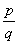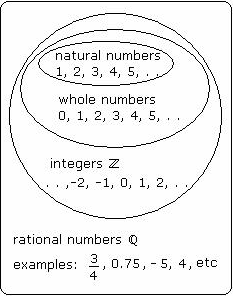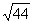## Definition Of Rational Numbers

A Rational Number is a real number written as a ratio of integers with a non-zero denominator.

Rational numbers are indicated by the symbol Q
Rational number is written inform, where p and q are integers and q is a non-zero denominator.
All the repeating or terminating decimal numbers are rational numbers.
Rational numbers are the subset of real numbers.

### Examples of Rational Numbers

3/5,10.3,0.6,12/5,3/4 - All these are examples of rational numbers as they terminate.### Solved Example on Rational Numbers

#### Ques: Identify a number that is a rational number but not an integer.

##### Choices:

A.B. 10.3
C. - 3.523...
D. -Step 2:= - 5 is a rational number and also an integer.
Step 3:cannot be expressed as a quotient of two integers, so it is not a rational number.